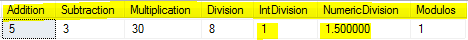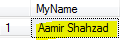### What Arithmetic Operators are available in SQL Server - SQL Server / TSQL Part 129

Arithmetic Operators are used to perform mathematical operations such as Addition, Subtraction, Multiplication and Division etc.

1 ) + will be used for Addition
2) - will be used for Subtraction
3) * will be used for Multiplication
4) / will be used for Division
5) % will be used for Modulo ( Returns the integer remainder of a division)

Let's write our query to perform these operations

```Select
5-2 as Subtraction,
5*6 as Multiplication,
40/5 as Division,
6/4 as IntDivision,
6/4.0 as NumericDivision,
7%2 as Modulos```How to perform Arithmetic Operations in SQL Server - SQL Server / TSQL Tutorial

Noticed that when you divided 6/4 both integers, the output returned will be integer. In our case it returned 1. To get your output in decimals ,At least one of dividend or divisor need to be numeric(decimal).

I used static values for arithmetic operations. you can multiple columns, make sure they are numeric data types.

Addition ( + )  can also be used to concatenate the string in TSQL.

`Select 'Aamir ' + 'Shahzad' as MyName`How to use Addition Sign to perform Concatenation in SQL Server - SQL Server / TSQL Tutorial

The Addition and Subtraction signs can also be used to perform arithmetic operations on datetime and smalldatetime values.

Let's say that if I would like to add or subtract few days from Current date, I can use below query.

```Select GETDATE()+5 AS Add5eDaystoCurrentDate,
GETDATE()-30 AS Subtract30DaysfromCurrentDate```

#### 1 comment:

1.